# 机器学习-吴恩达：学习笔记及总结（5）

## Coursera | Machine Learning | Andrew Ng

Thistledown    March 14, 2020

Course Link ：Week 5 - Neural Networks: Learning

## 14 Cost Function and Backpropagation

#### 14.1 Cost Function

• Neural Network (Classification)

• Training Set: $\lbrace \left( x^{(1)}, y^{(1)} \right), \left( x^{(2)}, y^{(2)} \right), \dots, \left( x^{(m)}, y^{(m)} \right) \rbrace$
• $L$ = total number of layers in the network
• $s_l$ = number of units (not counting bias unit) in layer $l$
• $K$ = number of output units/classes
• Binary classification: $y = 0 \text{ or } 1$ (1 output unit)
• Multi-class classification (K classes): $y \in \mathbb{R}^K$ (K output units)
• Cost function

• Logistic regression:

• Neural network: ($h_\Theta(x)\in \mathbb{R}^K, \left( h_\Theta\left(x\right) \right)_k = k^{\text{th}} \text{ output}$)

• We have added a few nested summations to account for our multiple output nodes.
• In the first part of the equation, before the square brackets, we have an additional nested summations that loops through the number of output nodes.
• In the regularization part, after the square brackets, we must account for multiple matrices. The number of columns in our current theta matrix is equal to the number of nodes in our current layer (including the bias unit). The number of rows in our current theta matrix is equal to the number of nodes in the next layer (excluding the bias unit). As before with logistic regression, we square every term.
• Note:

• The double sum simply adds up the logistic regression costs calculated for each cell in the output layer
• The triple sum simply adds up the squares of all the individual $\Theta$(s) in the entire network
• The $i$ in the triple sum does not refer to training example $i$

#### 14.2 Backpropagation Algorithm

• Backpropagation is a neural-network terminology for minimizing our cost function, just like what we were doing with gradient descent in logistic and linear regression.

• Our goal is to compute $\mathop{\text{min}}_\limits{\Theta}J(\Theta)$

• That is, we want to minimize our cost function $J$ using an optimal set of parameters in theta. In this section we’ll look at the equations we use to compute the partial derivative of $J(\Theta)$: $\frac{\partial}{\partial\Theta_{i,j}^{(l)}}J(\Theta)$

• To do so, we use the following algorithm: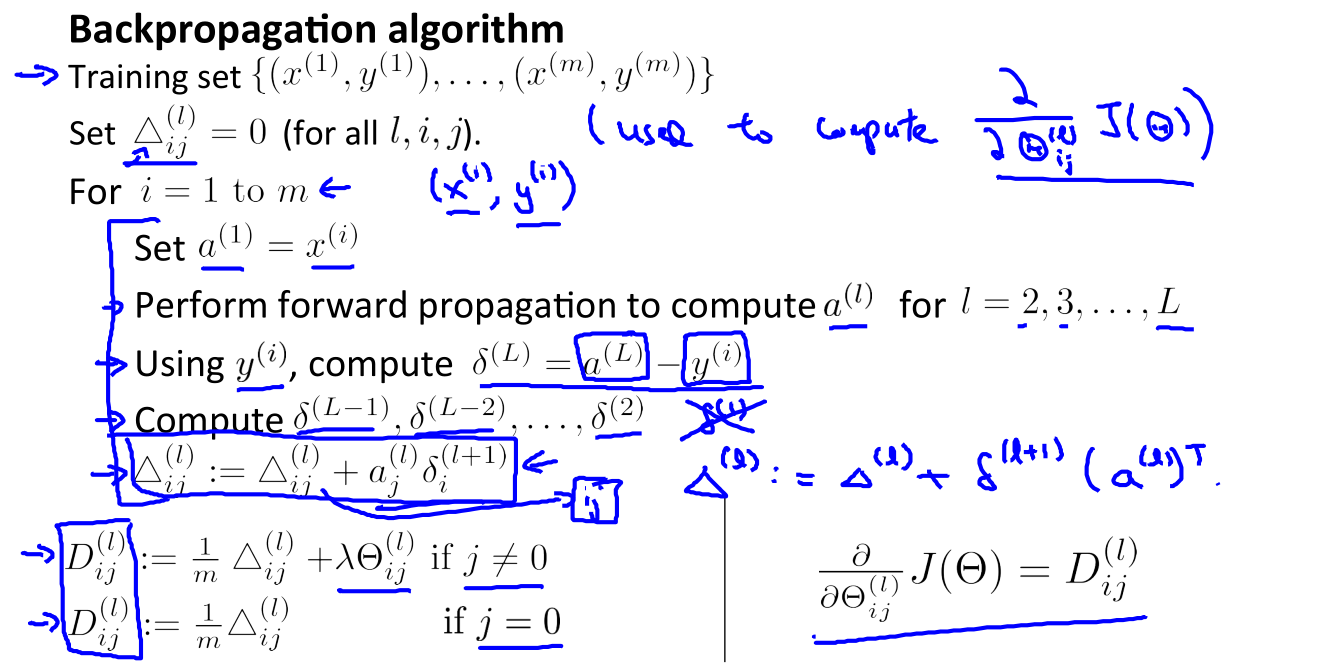• Backpropagation Algorithm

• Given training set $\lbrace \left( x^{(1)}, y^{(1)} \right), \left( x^{(2)}, y^{(2)} \right), \dots, \left( x^{(m)}, y^{(m)} \right) \rbrace$

• Set $\Delta_{i,j}^{(l)}:=0$ for all $(l,i,j)$ (hence you end up having a matrix full of zeros)

• For training example $i=1\text{ to } m$

• Set $a^{(1)} := x^{(i)}$

• Perform forward propagation to compute $a^{(l)}$ for $l = 2, 3, \dots,L$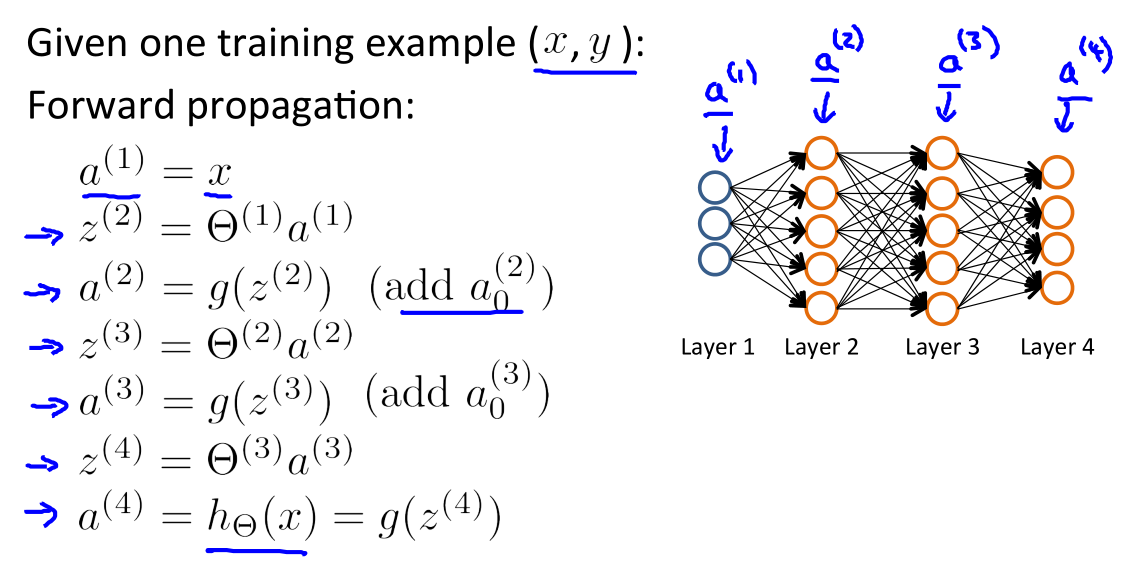• Using $y^{(i)}$, compute $\delta^{(L)}=a^{(L)}-y^{(L)}$

• $L$ is our total number of layers
• $a^{(L)}$ is the vector of outputs of the activation units for the last layer
• $\delta_j^{(l)}$ = “error” of node $j$ in layer $l$
• So our “error values” for the last layer are simply the differences of our actual results in the last layer and the correct outputs in $y$.
• To get the delta values of the layers before the last layer, we can use an equation that steps us back from right to left.

• Compute $\delta^{(L-1)}, \delta^{(L-2)}, \dots, \delta^{(2)}$ using

• The delta values of layer $l$ are calculated by multiplying the delta values in the next layer with the theta matrix of layer $l$

• We then element-wise multiply that with a function called $g^\prime$, which is the derivative of the activation function $g$ evaluated with the input values given by $z^{(l)}$

• The $g^\prime$ derivative terms can also be written out as:

• $\Delta^{(l)}_{i,j}:=\Delta^{(l)}_{i,j}+a_j^{(l)}\delta_i^{(l+1)}$ or with vectorization, $\Delta^{(l)}:=\Delta^{(l)}+\delta^{(l+1)}\left(a^{(l)}\right)^T$

• Hence we update our new $\Delta$ matrix.

The capital-delta matrix $D$ is used as an “accumulator” to add up our values as we go along and eventually compute our partial derivative. Thus we get $\frac \partial {\partial \Theta_{ij}^{(l)}} J(\Theta) = D_{i,j}^{(l)}$

#### 14.3 Backpropagation Intuition

• Recall that the cost function for a neural network is:

• If we consider simple non-multiclass classification ($k=1$) and disregard regularization, the cost is computed with:

• Intuitively, $\delta_j^{(l)}$ is the “error” for $a_j^{(l)}$ (unit $j$ in layer $l$). More formally, the delta values are actually the derivative of the cost function:

• Recall that our derivative is the slope of a line tangent to the cost function, so the steeper the slope the more incorrect we are. Let us consider the following neural network below and see how we could calculate some $\delta_j^{(l)}$: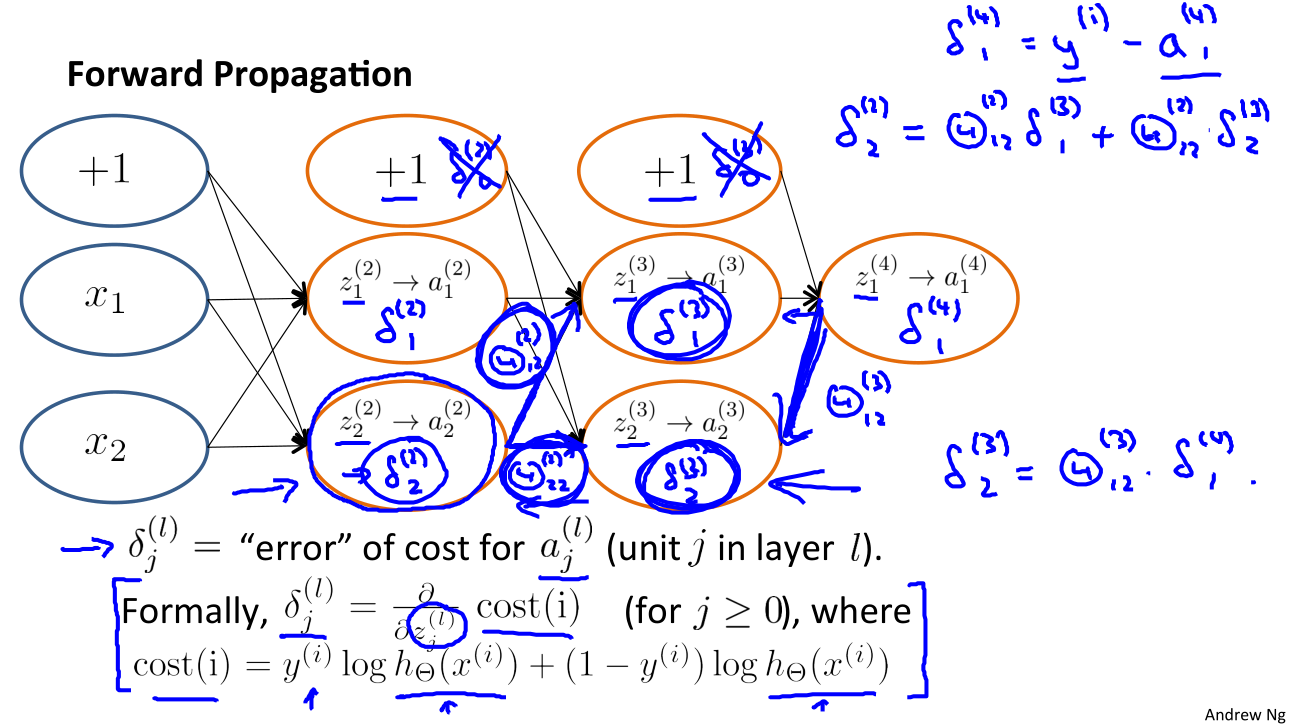In the image above, to calculate $\delta_2^{(2)}$, we multiply the weights $\Theta_{12}^{(2)}$ and $\Theta_{22}^{(2)}$ by their respective $\delta$ values found to the right of each edge. So we get $\delta_2^{(2)} = \Theta_{12}^{(2)}*\delta_1^{(3)} + \Theta_{22}^{(2)}*\delta_2^{(3)}$. To calculate every single possible $\delta_j^{(l)}$, we could start from the right of our diagram. We can think of our edges as our $\Theta_{ij}$. Going from right to left, to calculate the value of $\delta_j^{(l)}$, you can just take the over all sum of each weight times the $\delta$ it is coming from. Hence, another example would be $\delta_2^{(3)} = \Theta_{12}^{(3)}*\delta_1^{(4)}$.

## 15 Backprogation in Practice

#### 15.1 Implementation Note: Unrolling Parameters

• With neural networks, we are working with sets of matrices:

• In order to use optimizing functions such as fminunc(), we will want to “unroll” all the elements and put them into one vector.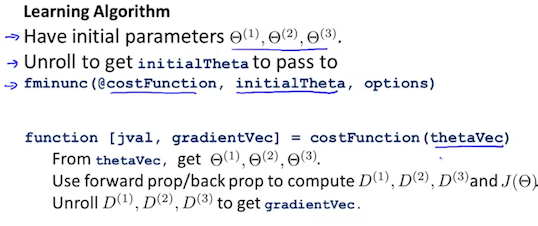• Gradient Checking will assure that our backpropagation works as intended. We can approximate the derivative of our cost function with:

• With multiple theta matrices, we can approximate the derivative with respect to $\Theta_j$ as follows:

• A small value for $\epsilon$ such as $\epsilon = 10^{-4}$, gurantees that the math works out properly. If the value for $\epsilon$ is too small, we can end up with numerical problems.

• Hence, we are only adding or subtracting epsilon to $\Theta_j$ matrix.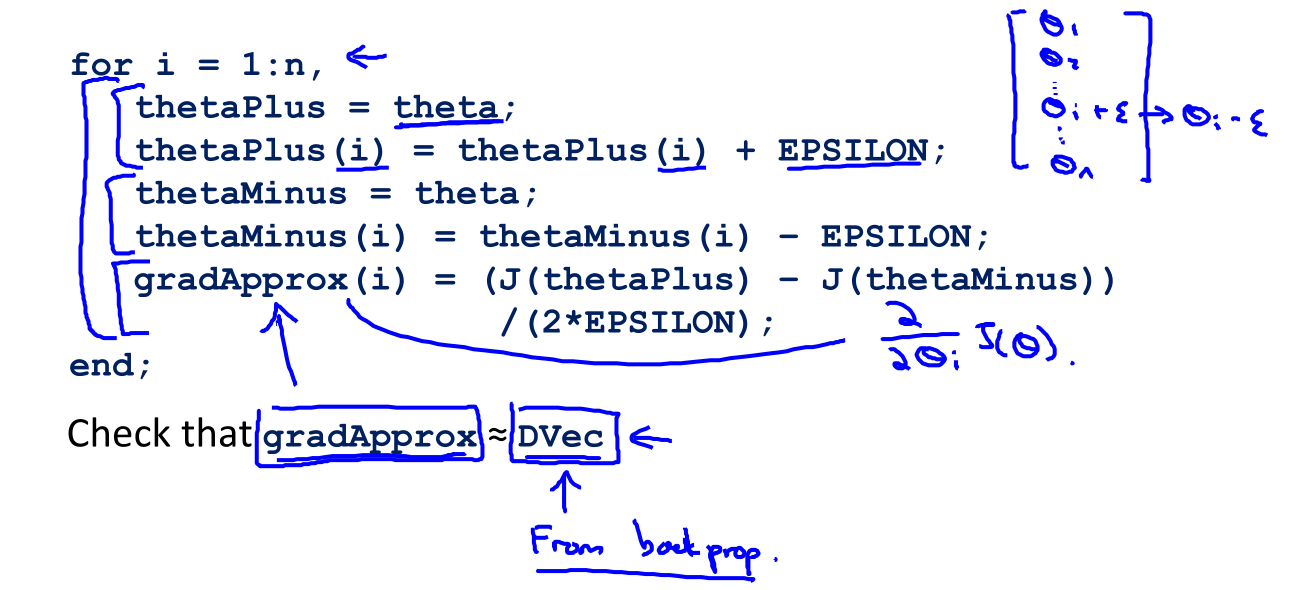We previously saw how to calculate the $\text{deltaVector}$. So once we compute our $\text{gradApprox}$ vector, we can check that $\text{gradApprox$\approx$deltaVector}$

• Implementation Note:

• Implement backprop to compute $\text{DVec}$ (Unrolled $D^{(1)}, D^{(2)}, D^{(3)}$).
• Implement numerical gradient check to compute $\text{gradApprox}$.
• Make sure they give similar values.
• Turn off gradient checking. Using backprop code for learning.
• Important: Be sure to disable your gradient checking code before training your classifier. The code to compute $\text{gradApprox}$ can be very slow.

#### 15.3 Random Initialization

• Initializing all theta weights to zero does not work with neural networks. When we backpropagete, all nodes will update to the same value repeatedly. Instead wo can randomly initialize our weights for our $\Theta$ matrices using the following method: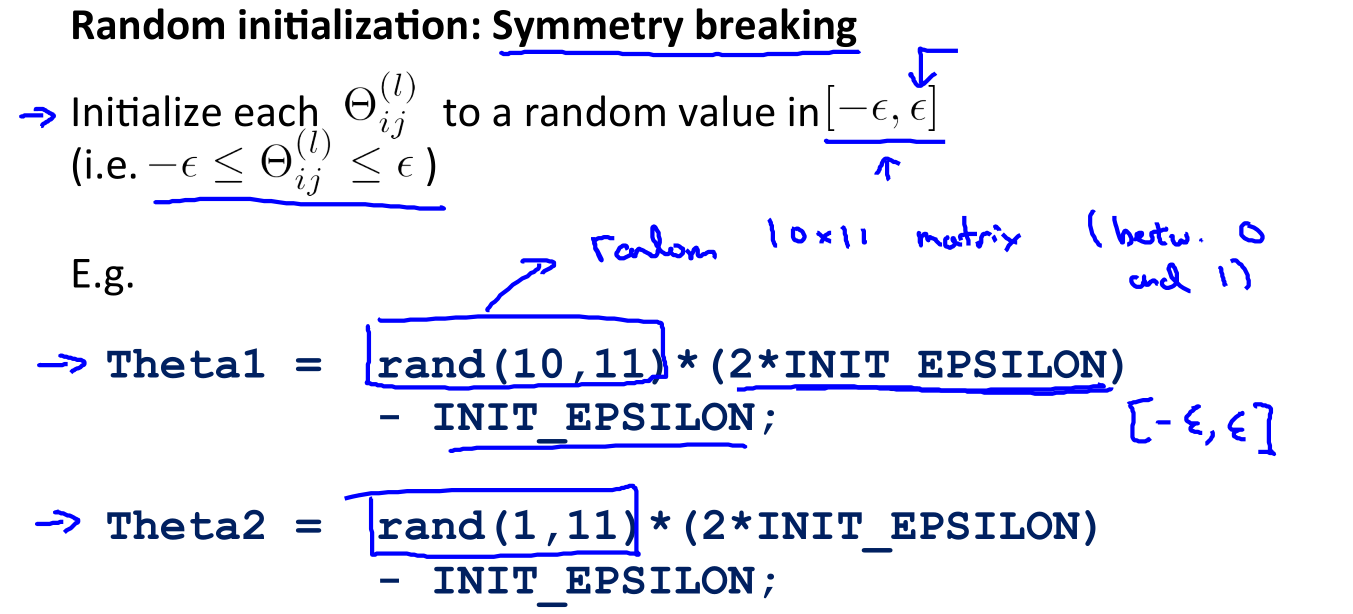• Hence, we initialize each $\Theta_{ij}^{(l)}$ to a random value between $[-\epsilon, \epsilon]$. Using the above formula gurantees that we get the desired bound. The same procedure applies to all the $\Theta$’s.
• Note: the epsilon used above is unrelated to the epsilon from Gradient Checking.

#### 15.4 Putting It Together

• First, pick a network architecture. Choose the layout of your neural network, including how many hidden units in each layer and how many layers in total you want to have.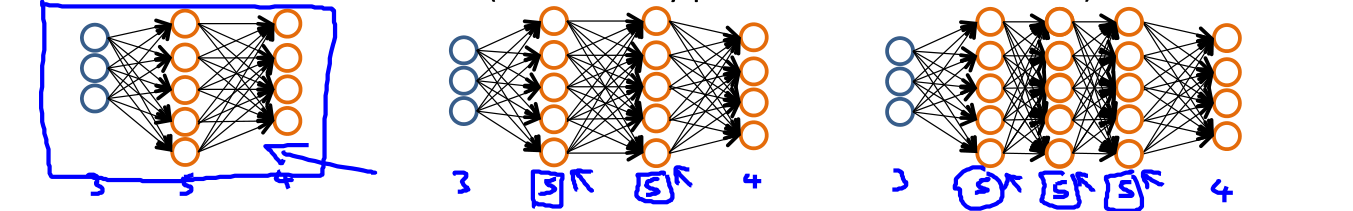• Number of input units = dimension of features $x^{(i)}$
• Number of output units = number of classes
• Number of hidden units per layer = usually more the better (must balance with cost of computation as it increases with more hidden units)
• Defaults: 1 hidden layer. If you have more than 1 hidden layer, then it is recommended that you have the same number of units in every hidden layer.
• Training a neural network
• Randomly initialize the weights.
• Implement forward propagation to get $h_\Theta\left(x^{(i)}\right)$ for any $x^{(i)}$.
• Implement the cost function.
• Implement backpropagation to compute partial derivatives.
• Use gradient descent or a built-in optimization function to minimize the cost function with the weights in theta.
• When we perform forward and back propagation, we loop on every training example: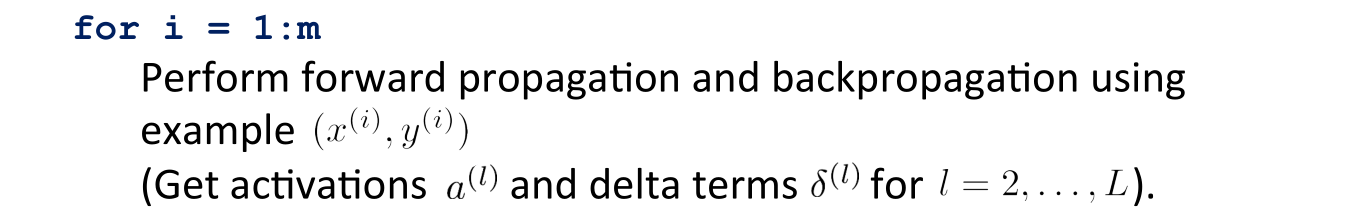• The following image gives us an intuition of what is happening as we are implements our neural network: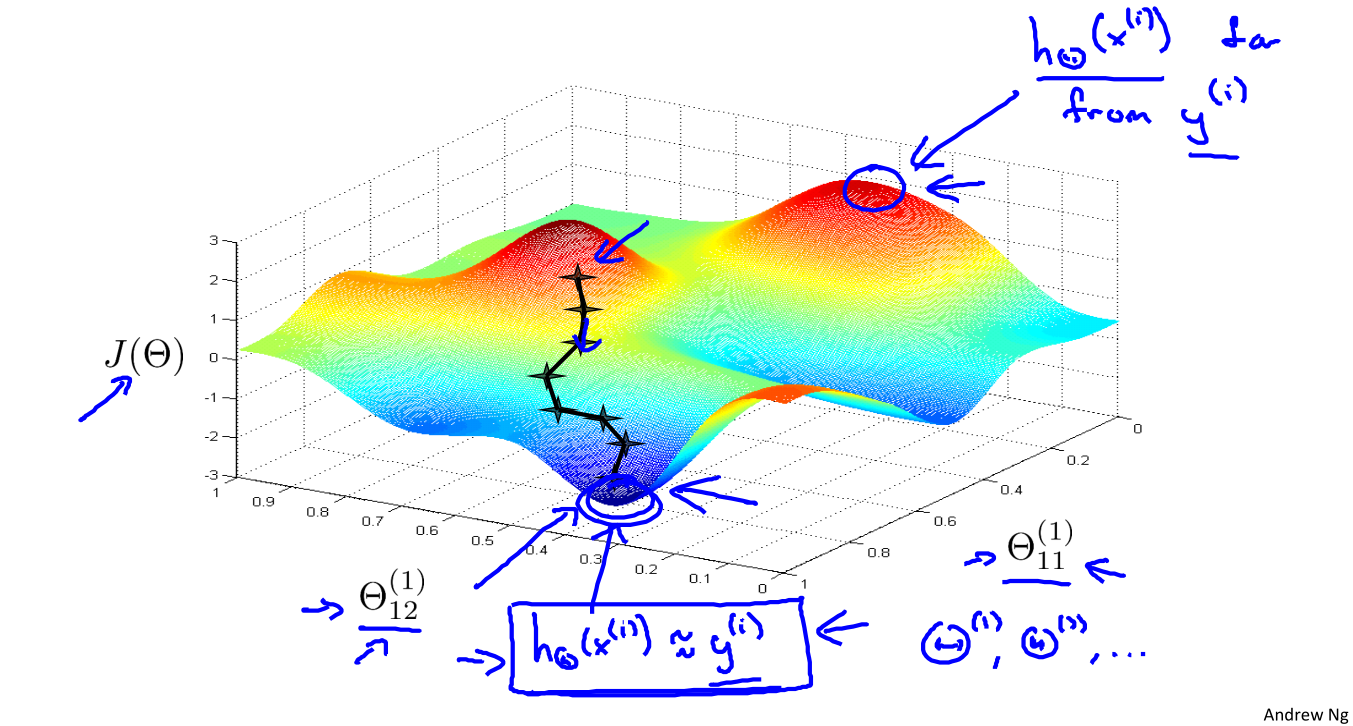Ideally, you want $h_\Theta\left(x^{(i)}\right) \approx y^{(i)}$. This will minimize our cost function. However, keep in mind that $J(\Theta)$ is not convex and thus we can end up in a local minimum instead.

## Ex4: Neural Networks Learning👨‍💻

See this exercise on Coursera-MachineLearning-Python/ex4/ex4.ipynb

#### Ex4.1&4.2 Neural Networks&Backpropagation

Instruction:

In the previous exercise, you implemented feedforward propagation for neural networks and used it to predict handwritten digits with the weights we provided. In this exercise, you will implement the backpropagation algorithm to learn the parameters for the neural network.

Code:

1
2
3
4
5
6
7
8
9
10
11
12
13
14
15
16
17
18
19
20
21
22
23
24
25
26
27
28
29
30
31
32
33
34
35
36
37
38
39
40
41
42
43
44
45
46
47
48
49
50
51
52
53
54
55
56
57
58
59
60
61
62
63
64
65
66
67
68
69
70
71
72
73
74
75
76
77
78
79
80
81
82
83
84
85
86
87
88
89
90
91
92
93
94
95
96
97
98
99
100
101
102
103
104
105
106
107
108
109
110
111
112
113
114
115
116
117
118
119
120
121
122
123
124
125
126
127
128
129
130
131
132
133
134
135
136
137
138
139
140
141
142
143
144
145
146
147
148
149
150
151
152
153
154
155
156
157
158
159
160
161
162
163
164
165
166
167
168
169
170
171
172
173
174
175
176
177
178
179
180
181
182
183
184
185
186
187
188
189
190
191
192
193
194
195
196
197
198
199
200
201
202
203
204
205
206
207
208
209
210
211
212
213
214
215
216
217
218
219
220
221
222
223
224
225
226
227
228
229
230
231
232
233
234
235
236
237
238
239
240
241
242
243
244
245
246
247
248
249
250
251
252
253
254
255
256
257
258
259
260
261
262
263
264
265
266
267
268
269
270
271
272
273
274
275
import numpy as np
import matplotlib.pyplot as plt
import scipy.io as scio
import scipy.optimize as opt

''' Part 0: Functions and Parameters '''
X, y = data['X'], data['y']
m = len(y)
return X, y, m

# Randomly select several data points to display
def randomDisplay(data, num=100, cmap='binary', transpose=True):
sample = data[np.random.choice(len(data), num)]
size, size1 = int(np.sqrt(num)), int(np.sqrt(sample.shape))
fig0, ax0 = plt.subplots(nrows=size, ncols=size, sharex=True, sharey=True, figsize=(8, 8))
order = 'F' if transpose else 'C'
for i in range(size):
for j in range(size):
ax0[i, j].imshow(sample[size * i + j].reshape([size1, size1], order=order), cmap=cmap)
plt.xticks(np.array([]))
plt.yticks(np.array([]))
plt.suptitle(str(num)+' examples from the dataset', fontsize=24)

# Implements the neural network cost function for a two layer neural network
# which performs classification
def nnCostFunction(nn_params, input_layer_size, hidden_layer_size,
num_labels, X, y, reg_param):
# Reshape nn_params back into the parameters Theta1 and Theta2,
# the weight matrices for our 2 layer neural network
Theta1 = nn_params[:hidden_layer_size * (input_layer_size + 1)].\
reshape([hidden_layer_size, (input_layer_size + 1)], order='F')
Theta2 = nn_params[hidden_layer_size * (input_layer_size + 1):].\
reshape([num_labels, (hidden_layer_size + 1)], order='F')

# Setup some useful variables
m = X.shape

# Feedforward Propagation
a1 = np.c_[np.ones([X.shape, 1]), X]
z2 = np.dot(a1, Theta1.T)
a2 = np.c_[np.ones([z2.shape, 1]), sigmoid(z2)]
z3 = np.dot(a2, Theta2.T)
a3 = sigmoid(z3)

y = np.eye(num_labels)[y.reshape(-1) - 1]

cost1 = - np.sum((np.log(a3) * y) + np.log(1 - a3) * (1 - y)) / m
cost2 = 0.5 * reg_param * (np.sum(np.square(Theta1[:, 1:])) +
np.sum(np.square(Theta2[:, 1:]))) / m
cost = cost1 + cost2

# Backpropagation
delta3 = a3 - y
delta2 = np.dot(delta3, Theta2)[:, 1:] * sigmoidGradient(z2)
Delta1, Delta2 = np.dot(delta2.T, a1), np.dot(delta3.T, a2)

Theta1_grad[:, 1:] += reg_param * Theta1[:, 1:] / m
Theta2_grad[:, 1:] += reg_param * Theta2[:, 1:] / m

# Sigmoid function
def sigmoid(z):
return 1 / (1 + np.exp(-z))

# sigmoidGradient() returns the gradient of the sigmoid function evaluated at z
return sigmoid(z) * (1 - sigmoid(z))

# Randomly initialize the weights of a layer with L_in incoming connections and L_out outgoing connections
def randInitWeights(L_in, L_out, epsilon_init=0.12):
# epsilon_init = np.sqrt(6) / np.sqrt(L_in + L_out)
W = np.random.rand(L_out, 1 + L_in) * 2 * epsilon_init - epsilon_init
return W

# Initialize the weights of a layer with fan_in incoming connections and fan_out
# outgoing connections using a fixed strategy, this will help you later in debugging
def debugInitWeights(fan_in, fan_out):
# Initialize W using "sin", this ensures that W is always of the same values
# and will be useful for debugging
W = np.sin(np.arange(1, 1 + (1 + fan_in) * fan_out)) / 10.0
W = W.reshape(fan_out, 1 + fan_in, order='F')
return W

# Computes the gradient using "finite differences" nd gives us
# a numerical estimate of the gradient.
perturb = np.diag(e * np.ones(nn_params.shape))
for i in range(nn_params.size):
loss1, _ = costFunc(nn_params - perturb[:, i])
loss2, _ = costFunc(nn_params + perturb[:, i])
numgrad[i] = (loss2 - loss1) / (2 * e)

# Creates a small neural network to check the backpropagation gradients
input_layer_size = 3
hidden_layer_size = 5
num_labels = 3
m = 5

# We generate some 'random' test data
Theta1 = debugInitWeights(input_layer_size, hidden_layer_size)
Theta2 = debugInitWeights(hidden_layer_size, num_labels)
# Reusing debugInitWeights to generate X
X = debugInitWeights(input_layer_size - 1, m)
y = np.arange(1, 1 + m) % num_labels

# Unroll parameters
nn_params = np.r_[Theta1.ravel(order='F'), Theta2.ravel(order='F')]

# Short hand for cost function
costFunc = lambda p: nnCostFunction(p, input_layer_size, hidden_layer_size, num_labels, X, y, reg_param)

# Visually examine the two gradient computations.
# The two columns you get should be very similar
if (visually_examine):
print(' - The above two columns you get should be very similar.')

# Evaluate the norm of the difference between two solutions.
# If you have a correct implementation, and assuming that you used EPSILON = 1e-4
# in 'computeNumericalGradient()', then diff below should be less that 1e-9
print(' - If your backpropagation implementation is correct, \n'
' - then the relative difference will be small (less than 1e-9).\n'
' - Relative Difference:', diff)

# Predict the label of an input given a trained neural network
def predict(theta1, theta2, X):
a1 = np.c_[np.ones([X.shape, 1]), X]
z2 = np.dot(a1, theta1.T)
a2 = np.c_[np.ones([z2.shape, 1]), sigmoid(z2)]
z3 = np.dot(a2, theta2.T)
a3 = sigmoid(z3)
pred = np.argmax(a3, axis=1)
return pred + 1

# Visualize what the representations captured by the hidden units
def plotHiddenUnits(X, figsize=(8, 8)):
if X.ndim == 2:
m, n = X.shape
elif X.ndim == 1:
m, n = 1, X.size
X = X[None] # Promote to a 2-D array
else:
raise IndexError('Input X should be 1 or 2 dimensional.')

size = int(np.sqrt(n))
rows = cols = int(np.sqrt(m))

fig1, ax1 = plt.subplots(rows, cols, sharex=True, sharey=True, figsize=figsize)
ax1 = [ax1] if m == 1 else ax1.ravel()
for i, ax in enumerate(ax1):
ax.imshow(X[i].reshape([size, size], order='F'), cmap='Greys')
plt.xticks(np.array([]))
plt.yticks(np.array([]))
plt.suptitle('Visualization of Hidden Units', fontsize=24)

# Setup the parameters
input_layer_size = 400  # 20x20 Input Images of Digits
hidden_layer_size = 25  # 25 hidden units
num_labels = 10         # 10 labels, form 1 to 10 (mapping '0' to label '10')

# Randomly select 100 data points to display
randomDisplay(X, 100, transpose=True)

# Load the weights into variables Theta1 and Theta2
Theta1, Theta2 = weights['Theta1'], weights['Theta2']

# Unroll parameters
nn_params = np.r_[Theta1.ravel(order='F'), Theta2.ravel(order='F')]

''' Part 3: Compute Cost (Feedforward) '''
print('3. Feedforward Using Neural Network ...')
# Set weight regularization parameter to 0 (i.e. no regularization)
cost, _ = nnCostFunction(nn_params, input_layer_size, hidden_layer_size,
num_labels, X, y, reg_param=0)
print(' - Cost at parameters (loaded form ex4weights.mat): ', cost)
print(' - (This value should be about 0.287629.)\n')

''' Part 4: Implement Regularization '''
print('4. Checking Cost Function (w/ Regularization) ...')
# Weight regularization parameter (we set this to 1 here)
cost, _ = nnCostFunction(nn_params, input_layer_size, hidden_layer_size,
num_labels, X, y, reg_param=1)
print(' - Cost at parameters (loaded form ex4weights.mat): ', cost)
print(' - (This value should be about 0.383770.)\n')

''' Part 5: Sigmoid Gradient '''
g = sigmoidGradient(np.array([-1, -0.5, 0, 0.5, 1]))
print(' - Sigmoid gradient evaluated at [-1, -0.5, 0, 0.5, 1]:\n -', g)

''' Part 6: Initializing Parameters '''
print('\n6. Initializing Neural Network Parameters ...')
init_Theta1 = randInitWeights(input_layer_size, hidden_layer_size)
init_Theta2 = randInitWeights(hidden_layer_size, num_labels)

# Unroll parameters
init_nn_params = np.r_[init_Theta1.ravel(order='F'), init_Theta2.ravel(order='F')]

''' Part 7: Implement Backpropagation '''
print('\n7. Checking Backpropagation ...')

''' Part 8: Implement Regularization '''
print('\n8. Checking Backpropagation (w/ Regularization) ...')
debug_lambda = 3.0

debug_cost, _ = nnCostFunction(nn_params, input_layer_size, hidden_layer_size,
num_labels, X, y, debug_lambda)
print(' - Cost at (fixed) debugging parameters (w/ lambda = ', debug_lambda, '):',
debug_cost, '\n - (for lambda = 3, this value should be about 0.576051)\n')

''' Part 9: Training Neural Network '''
print('9. Training Neural Network ...')
# After you have completed the assignment, change the MaxIter
# to a larger value to see how training helps
options = {'maxiter': 400}

# You should also try different values of lambda
train_lambda = 3.0

# Create "short hand" for the cost function to be minimized
costFunc = lambda p: nnCostFunction(p, input_layer_size, hidden_layer_size,
num_labels, X, y, train_lambda)

# Now, 'costFunc' is a function that takes in only one argument
# (the neural network parameters)
res = opt.minimize(costFunc, init_nn_params, jac=True, method='TNC', options=options)
nn_params = res.x

# Obtain Theta1 and Theta2 back from nn_params
Theta1 = nn_params[:hidden_layer_size * (input_layer_size + 1)].\
reshape([hidden_layer_size, input_layer_size + 1], order='F')
Theta2 = nn_params[hidden_layer_size * (input_layer_size + 1):].\
reshape([num_labels, hidden_layer_size + 1], order='F')

# Implement predict
y_predict = predict(Theta1, Theta2, X)
print(' - When lambda = {:}, MaxIter = {:}, Training set accuracy = {:.2f}%\n'.
format(train_lambda, options['maxiter'], np.mean(y_predict == y.flatten()) * 100))

''' Part 10: Visualize Weights '''
print('10. Visualizing Neural Network ...\n')
plotHiddenUnits(Theta1[:, 1:])
plt.show()


Outputs:

• Console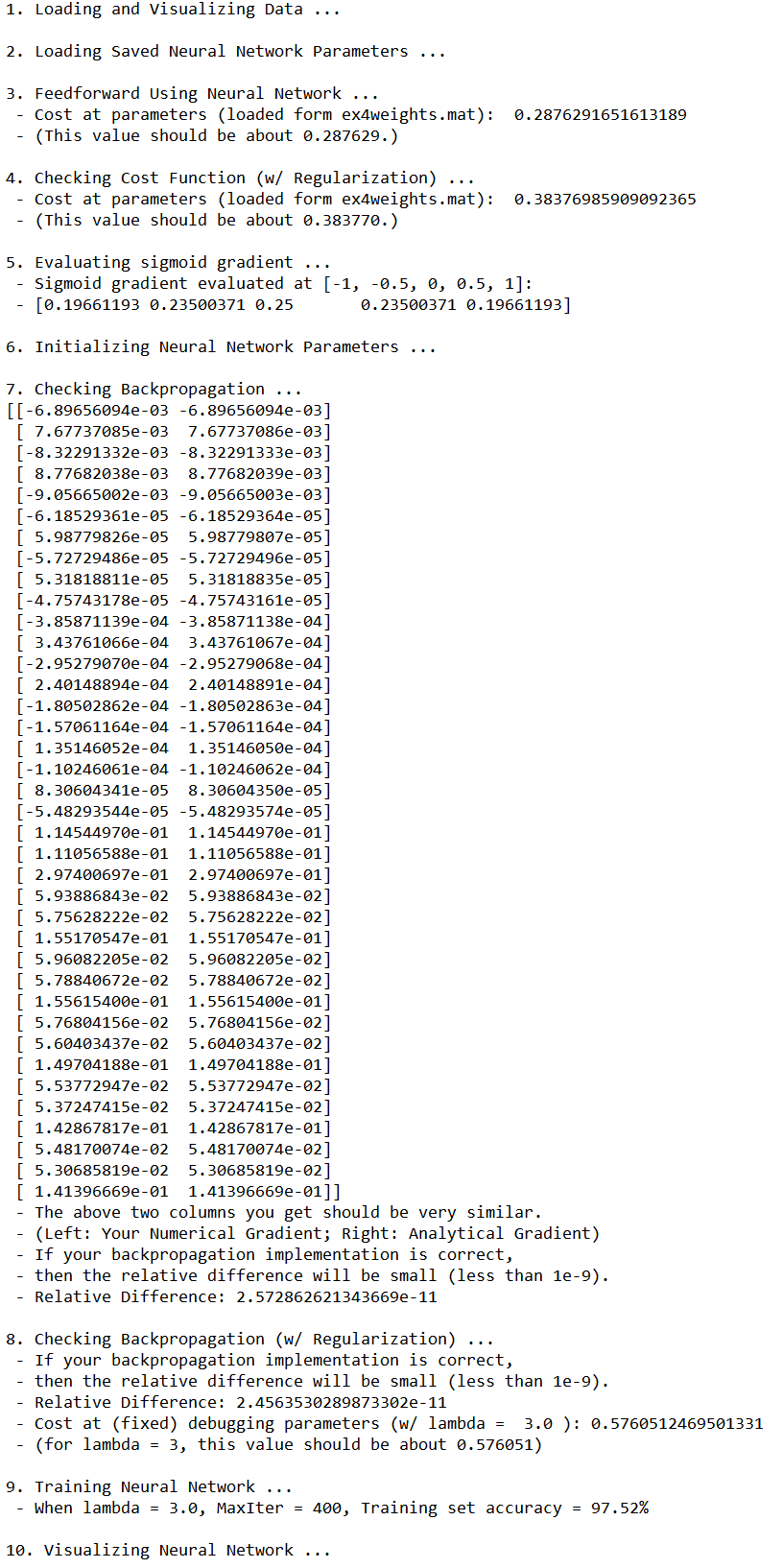• Randomly select 100 data points to display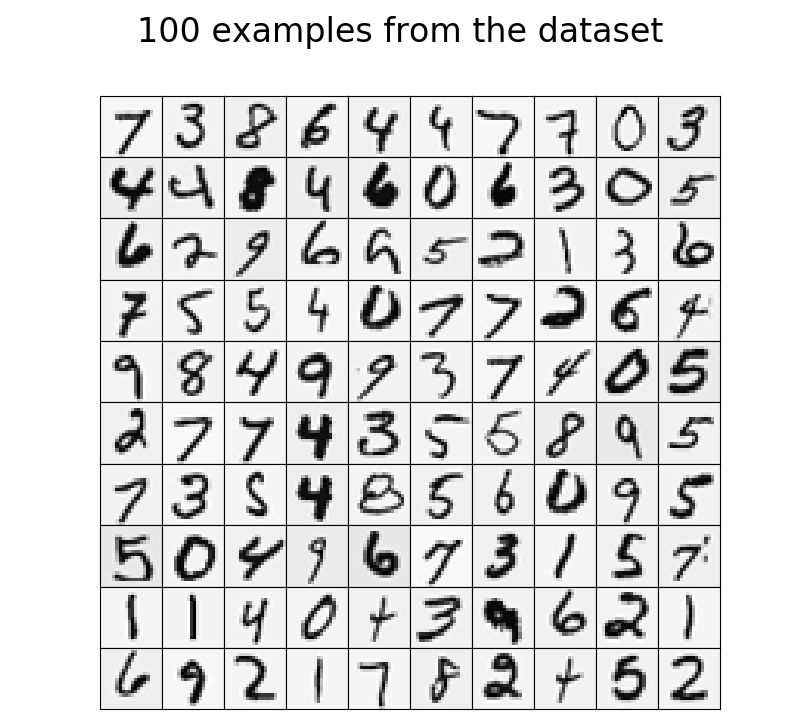• Visualization of hidden units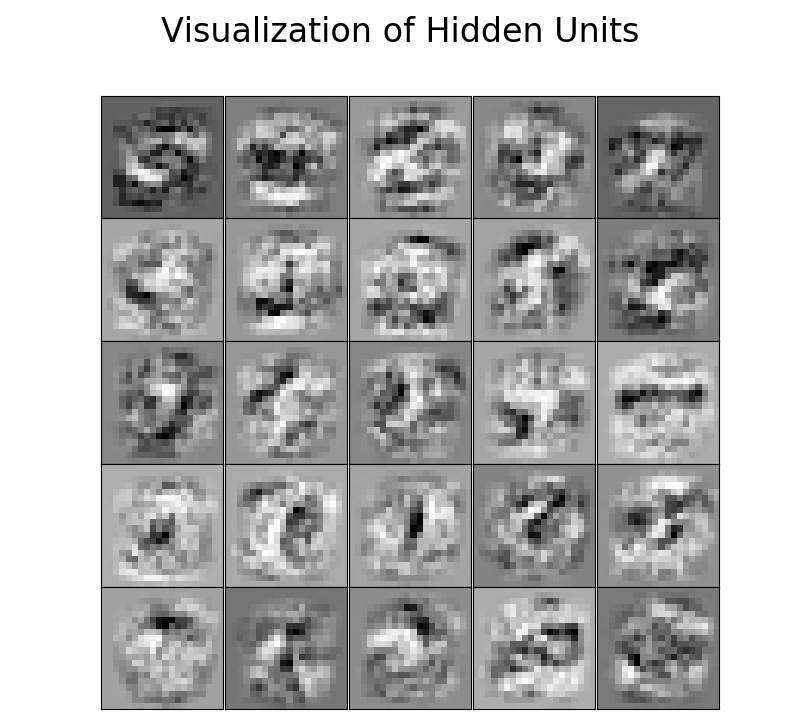Thistledown